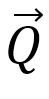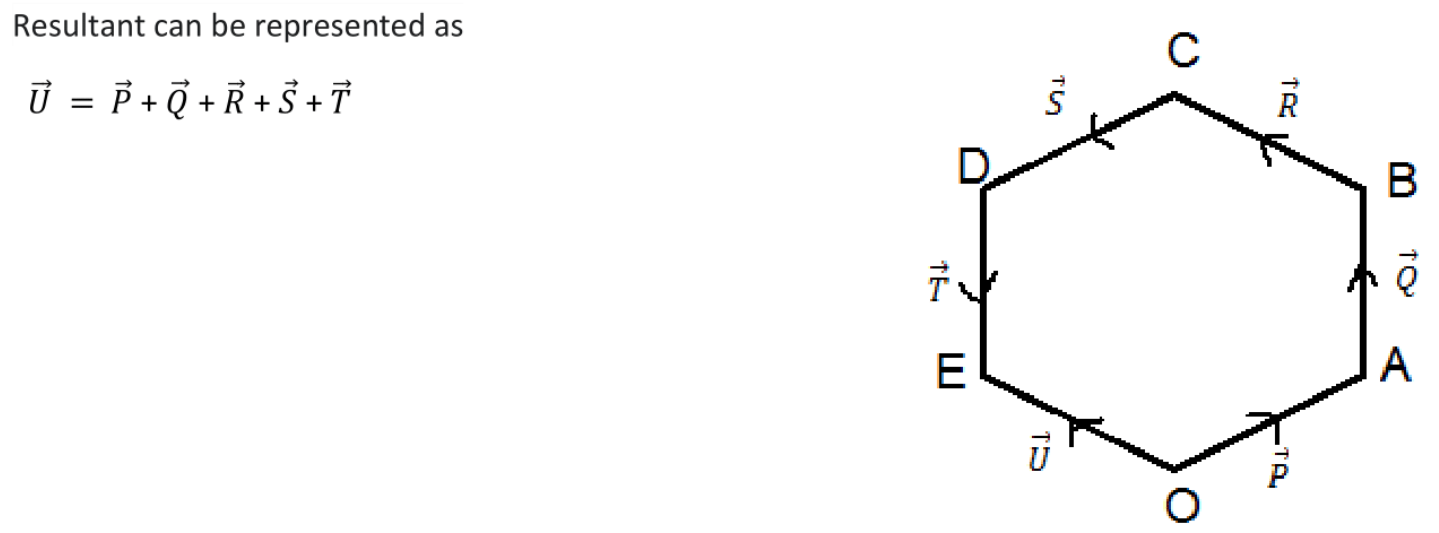# Vectors1.1 Scalars:- Those quantities which have magnitude only but no direction is called scalars. For example: area, volume, length, speed, current, electric charge, frequency, potential intensity or radiation, flux of field or vector, etc.

1.2 Vectors:- Those quantities which have both magnitude and direction and follow the law of vector addition is called vectors. For examples: impulse, force, gravity, displacement, magnetic moment, angular velocity, gradient of any quantity length element, area element, field intensity, etc.

­-Necessary conditions to be a vector: Must have magnitude and direction

-Sufficient condition to be a vector: Must obey the vector addition law.

1.3 Tensors:- Those physical quantities having no direction but at different magnitude have different direction. For examples: moment of inertia, stress, strain, refractive index, electric current, electrical conductivity, etc.

2.1 Types of vectors on major basis

i. Polar(or real) vector:- The vector having starting point(as in case of displacement) or point of application(as in the case of force) is called polar vector.

-They have natural direction i.e. observed easily.

For  examples: displacement, acceleration, impulse, force, etc.

ii. Axial(or pseudo) vector:- The vector represents rotation and are always along the axis of rotation in accordance with right hand screw or right hand thumb rule.

-They don’t have natural direction.

For examples: angular displacement, angular velocity, angular acceleration, torque, magnetic moment, etc.

Other special types of vector

i. Zero or null vector:- The vector having zero magnitude and indeterminant direction is called zero vector.

ii. Proper vector:- The vector having non-zero magnitude is called proper vector.

iii. Co-planer vector:- The vectors lying on the same plane or parallel plane is called co-planer vector.

iv.  Co-linear(or parallel) vector:- The vector having parallel rays or supports is called co-linear vector.

i) Like vector:- Parallel vector having same directions.

ii) Unlike vector:- Parallel vector having different directions.

v. Equal vector:- The vectors having equal magnitude and same direction is called equal vector.

vi. Negative vector:- The vectors having equal magnitude but opposite direction is called negative vector.

vii. Co-initial vector:- The vectors having same initial point or starting point is called co-initial vector.

viii. Co-terminal vector:- The vectors having same terminal or ending point is called co-terminal vector.

ix. Unit vector:- The vector having magnitude one is called unit vector.

-It represents the direction of the vector.

-And direction is given by,Whereis magnitude ofandis unit vector along the direction ofx.  Position vector:- The vectors which start from the origin to the coordinate system to the position of the vector is called position vector.

xi. Displacement vector:- The vector points from object initial position to its final position whose magnitude is equal to the straight line distance between them is known as displacement vector.

The process of combining two or more vectors in a single vector which is the resultant of them is called composition of vector.

3.2 Resultant of vectors or resultant vector

v  A single vector which produces same effect as is produced by individual vectors together is the resultant of the vectors.

There are three process of addition of vector:

1.      Triangular law of vector addition

2.      Parallelogram law of vector addition

3.      Polygon law of vector addition

1. Triangular law of vector addition

Statement: “If two vectorsandare represented in magnitude and direction by two adjacent sides of a triangle taken in same order, the resultant are=+is represented in both magnitude and direction by the third or closing side of the triangle in opposite vector.2. Parallelogram law of vector addition

Statement: If two vectors acting simultaneously at a point can be represented in both magnitude and direction by two adjacent sides of a parallelogram drawn from a point then their resultant is represented in both magnitude and direction by the diagonal of parallelogram drawn from that point.3. Polygonal law of vector addition

If a number of vectors are represented in both magnitude and direction by (n-1) sides of n sided polygon taken in the same order, then the nth side or closing side of this polygon in opposite order represents the resultant of them in both in magnitude and direction.5.1 Resolution of vector

The process of splitting a vector into two or more vectors in different direction in a plane such that their sum gives back the original vector is called resolution of vector.

The split vectors are called the components of the given vector.

Rectangular components of a vector

If the vector is split into two vectors in a plane at right angle to each other then they are said to be rectangular components of given vector.8.1 Relative velocity

Motion: Motion is the combined property of the the object under study & the observer.

It is always relative. There is no such thing like that is absolute in rest or in absolute motion.

Motion is measured with respect to the observer of reference frame.

Reference frame: It is the place or point with respect to which the motion is observed & measured. It may be co-ordinate system.

Two bodies A and B moving in a plane (two dimensional motion) may not have their motion along the some lines consequently their velocitiesandwill be inclined at some angle θ.